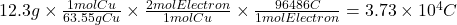## If 12.3 g of Cu is deposited at the cathode of an electrolytic cell after 5.50 h, what was the current used?​

Question

If 12.3 g of Cu is deposited at the cathode of an electrolytic cell after 5.50 h, what was the current used?​

in progress 0
6 months 2021-07-11T21:07:04+00:00 1 Answers 10 views 0

1.88 A

Explanation:

Let’s consider the reduction of copper in an electrolytic cell.

Cu²⁺ + 2 e⁻ ⇒ Cu

We can calculate the charge used to deposit 12.3 g of Cu using the following relations.

• The molar mass of Cu is 63.55 g/mol.
• 1 mole of Cu is deposited when 2 moles of electrons circulate.
• 1 mole of electrons has a charge of 96486 C (Faraday’s constant).

The charge used is:We can convert 5.50 h to seconds using the conversion factor 1 h = 3600 s.

5.50 h × 3600 s/1 h = 1.98 × 10⁴ s

The current used is:

I = q/t = 3.73 × 10⁴ C/1.98 × 10⁴ s = 1.88 A